首页 / 初中数学 / 试卷选题

# 2010年高级中等学校招生全国统一考试数学卷（云南昭通）• 题型：未知
• 难度：未知（1）这次抽样调查了多少人？
（2）在扇形统计图中，最喜欢娱乐节目对应的圆心角比最喜欢戏曲节目对应的圆心角大90°，调查中最喜欢娱乐节目比最喜欢戏曲节目的多多少人？
（3）估计南充城区有100万人中最喜欢体育节目的有多少人？

• 题型：未知
• 难度：未知

（1）求k的取值范围．
（2）请选择一个k的负整数值，并求出方程的根．

• 题型：未知
• 难度：未知（1）求证：△ABD∽△CED．

• 题型：未知
• 难度：未知（1）如果竖直摆放5个圆柱形桶时，网球能不能落入桶内？
（2）当竖直摆放圆柱形桶多少个时，网球可以落入桶内？

• 题型：未知
• 难度：未知（1）求∠BAC的度数．
（2）将△ACD沿AC折叠为△ACF，将△ABD沿AB折叠为△ABG，延长FC和GB相交于点H．求证：四边形AFHG是正方形．

• 题型：未知
• 难度：未知（1）求抛物线的解析式．
（2）如图，抛物线 $y=-\frac{1}{2}{x}^{2}+bx+4$$x$轴和 $y$轴的正半轴分别交于点 $A$$B$$M$$AB$的中点， $\angle PMQ$$AB$的同侧以 $M$为中心旋转，且 $\angle PMQ＝45°$$MP$$y$轴于点 $C$$MQ$$x$轴于点 $D$．设 $AD$的长为 $m（m＞0）$，BC的长为 $n$，求 $n$$m$之间的函数关系式

（3）当m，n为何值时，∠PMQ的边过点F．

• 题型：未知
• 难度：未知

 A．B．1 C．D．• 题型：未知
• 难度：未知的值为(    )

 A．－1 B．－3 C．1 D．0

• 题型：未知
• 难度：未知

 A．0.10×106 B．1.08×105 C．0.11×106 D．1.1×105

• 题型：未知
• 难度：未知

 A．2 B．0 C．－2 D．以上都不对

• 题型：未知
• 难度：未知

 A．2 B．5 C．6 D．7

• 题型：未知
• 难度：未知

(1)线段的中点是线段的重心
(2)三角形的三条中线交于一点，这一点就是三角形的重心
(3)平行四边形的重心是它的两条对角线的交点
(4)三角形的重心是它的中线的一个三等分点

 A．一个 B．两个 C．三个 D．四个

• 题型：未知
• 难度：未知

• 题型：未知
• 难度：未知

 A．B．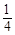C．D．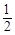• 题型：未知
• 难度：未知A．2B．C．1 D．2

• 题型：未知
• 难度：未知A．75(1＋)cm2 B．75(1＋)cm2 C．75(2＋)cm2 D．75(2＋)cm2

• 题型：未知
• 难度：未知

 A．ab＜0 B．ac＜0 C．当x＜2时，函数值随x增大而增大；当x＞2时，函数值随x增大而减小 D．二次函数y＝ax2＋bx＋c的图象与x轴交点的横坐标就是方程ax2＋bx＋c＝0的根• 题型：未知
• 难度：未知

• 题型：未知
• 难度：未知

• 题型：未知
• 难度：未知

• 题型：未知
• 难度：未知

$\odot O$中直径为4，弦 $AB＝2\sqrt{3}$，点 $C$是圆上不同于 $A$$B$的点，那么 $\angle ACB$度数为

• 题型：未知
• 难度：未知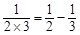…   …

• 题型：未知
• 难度：未知

 A．B．方程的解为C．D．• 题型：未知
• 难度：未知A                      B                     C                     D

• 题型：未知
• 难度：未知

 A．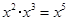B．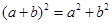C．D．• 题型：未知
• 难度：未知

Ｂ．抛掷一枚硬币，落地后正面朝上
Ｃ．当是实数时，Ｄ．长为的三条线段能围成一个三角形

• 题型：未知
• 难度：未知A．圆柱 B．球 C．正方体 D．长方体

• 题型：未知
• 难度：未知A．B．C．D．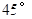• 题型：未知
• 难度：未知A．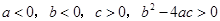B．C．D．• 题型：未知
• 难度：未知的相反数是__________．

• 题型：未知
• 难度：未知

• 题型：未知
• 难度：未知

• 题型：未知
• 难度：未知

• 题型：未知
• 难度：未知

• 题型：未知
• 难度：未知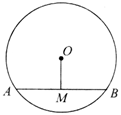• 题型：未知
• 难度：未知

• 题型：未知
• 难度：未知

• 题型：未知
• 难度：未知

• 题型：未知
• 难度：未知• 题型：未知
• 难度：未知

 户数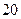节水量（立方米/每户）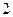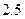• 题型：未知
• 难度：未知

（2）年我省已推广通过财政补贴节能灯万只，预计我省一年可节约电费亿元左右，减排二氧化碳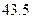万吨左右，请你估算一下全国一年大约可节约电费多少亿元？大约减排二氧化碳多少万吨？（结果精确到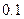• 题型：未知
• 难度：未知• 题型：未知
• 难度：未知（1）请你帮技术员算出水的深度（精确到米，参考数据）；
（2）就水的深度而言，平均每天水位下降必须控制在多少米以内，才能保证现有水量至少能使用天？（精确到米）

• 题型：未知
• 难度：未知• 题型：未知
• 难度：未知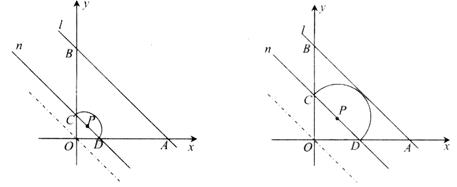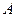两点的坐标；的函数关系式及自变量的取值范围；为何值时，半圆与直线相切？是否存在这样的值，使得半圆面积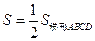？若存在，求出值，若不存在，说明理由．

• 题型：未知
• 难度：未知